# CBSE Class 10 Science Chapter 10 Light - Reflection and Refraction Notes

Visible light or light is an electromagnetic radiation found on the electromagnetic spectrum which enables us to see light. Objects reflect some colours of the spectrum when light falls on them. Learn in detail about light and discover other interesting concepts by exploring Class 10 notes for chapter 10 Light – Reflection and Refraction.

Chapter 10 Light – Reflection and Refraction Notes:-

Topics Covered In Chapter 10 Light – Reflection And Refraction:

Reflection Of Light

Spherical Mirrors

Image Formation By Spherical Mirrors

Representation Of Images Formed By Spherical Mirrors Using Ray Diagrams

Sign Convention For Reflection By Spherical Mirrors

Mirror Formula And Magnification

Refraction Of Light

Refraction Through A Rectangular Glass Slab

The Refractive Index

Refraction By Spherical Lenses

Image Formation By Lenses

Image Formation In Lenses Using Ray Diagrams

Sign Convention For Spherical Lenses

Lens Formula And Magnification

Power Of A Lens

Introduction To The Chapter

What is Light?

Light can be simply defined as the form of energy which helps us to see objects in dark. There are both natural and artificial sources of light. Sunlight is the best example for the natural sources of light. Reflection and refraction of light are the two main properties of light. Some common phenomena associated with lights are image formation by mirrors, the twinkling of stars, the beautiful colours of the rainbow, bending of light by a medium and so on.

Properties Of Light

• An electromagnetic wave so does not require any medium to travel
• Light tends to travel in a straight line
• Light has dual nature i.e., wave as well as particle
• Speed of light is maximum in vacuum. Its value is 3 x 108 m/s
• When light falls on a surface, the following happens

– Reflection

– Refraction

– Absorption

What is Reflection of light?

The natural phenomenon of bouncing back the light in the same medium on striking the surface of an object is termed as the Reflection of light. According to the laws of Reflection:

• The angle of reflection is always equal to the angle of incidence.
• All the three rays- incident, reflected ray and the normal lie in the same plane.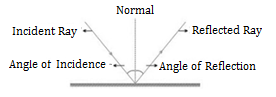Virtual and Real Image

Image is a point where at least two light rays actually meet or appear to meet.

 Real Image Virtual Image Formed when light rays actually meet Formed when light rays appear to meet Can be obtained on the screen Cannot be obtained on the screen inverted erect Example – image formed on cinema screen and formed by concave mirror Example – image formed by plane mirror or convex mirror

Image Formed By Plane Mirror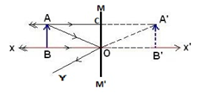Characteristics of Image formed by Plane Mirror

• Virtual and erect
• Size of image is equal to the size of object
• Image is formed as far behind the mirror as the object is in front of it
• Laterally inverted

Lateral Inversion – the right side of the object appears left side of the image and vice-versa

Application of Lateral Inversion

The word AMBULANCE is written in reverse direction so that it can be read correctly in the rearview mirror of vehicles going in front of it.

Spherical Mirrors

Mirrors whose reflecting surface is curved. There are two types of spherical mirrors, they are:

• Convex mirror
• Concave mirror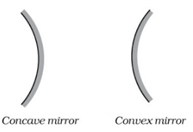Properties of Concave Mirror:

• Reflecting surface is curved inwards
• Converging mirror

Properties of Convex Mirror:

• Reflecting surface is curved outwards
• Diverging mirror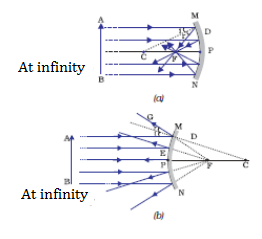(a) concave mirror (b) convex mirror

Common Terms for Spherical Mirrors

• Principal axis – the line joining pole and the centre of curvature
• Pole (P) – the centre of the spherical mirror
• Aperture (MN) – it is the effective diameter of the spherical mirror
• Centre of curvature (C) – The centre of the hollow glass sphere of which the mirror was a part
• Radius of curvature (R) – The distance between the pole and the centre of curvature
• Focus (F) – The point of principle axis where all the parallel light rays actually meet or appear to meet after reflection
• Focal Length (f) – The distance between the pole and the focus
• Relationship between focal length and the radius of curvature. f=R/2

Rules For Making Ray Diagrams By Spherical Mirror

(i) A ray passing to the principal axis, after reflection, will pass through the principal focus in case of a concave mirror or appear to diverge from the principal focus in case of a convex mirror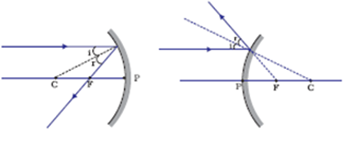(ii) A ray passing through the principal focus of a concave mirror or a ray which is directed towards the principal focus of a convex mirror, after reflection, will emerge parallel to the principal axis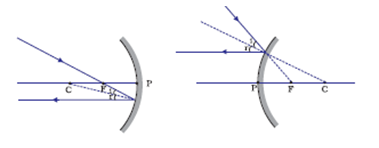(iii) A ray passing through the centre of curvature of a concave mirror or directed in the direction of the centre of curvature of a convex mirror, after reflection, is reflected back along the same path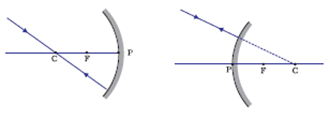(iv) A ray incident obliquely to the principal axis, towards a point P (pole of the mirror), on the concave mirror or a convex mirror, is reflected obliquely. The incident and reflected rays follow the laws of reflection at the point of incidence (point P), making equal angles with the principal axis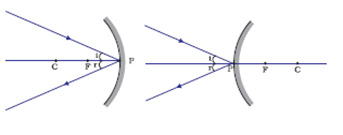Ray Diagrams For Images Formed by Concave Mirror

(i) When object is at infinity

Image Position – At ‘F’

Nature of image – Real, inverted

Size – Point sized or highly diminished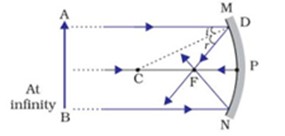(ii) When object is beyond ‘C’

Image Position – Between ‘F’ and ‘C’

Nature of image – Real, inverted

Size – Diminished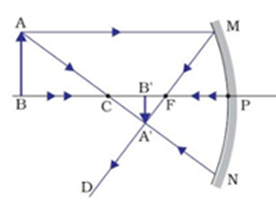(iii) When object is at ‘C’

Image Position – At ‘C’

Nature of image – Real, inverted

Size – Same size as that of object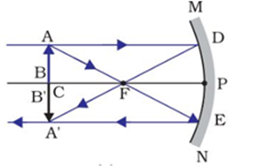(iv) When object is placed between ‘F’ and ‘C’

Image Position – Beyond ‘C’

Nature of image – Real, inverted

Size – Enlarged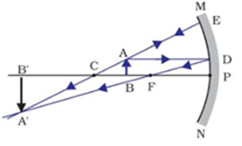(v) When object is placed at ‘F’

Image Position – At infinity

Nature of image – Real, inverted

Size – Highly enlarged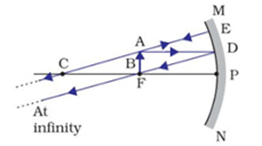(vi) When object is between ‘P’ and ‘F’

Image Position – Behind the mirror

Nature of image – Virtual, erect

Size – Enlarged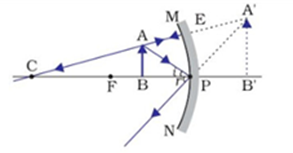Uses of Concave Mirror

• Used in torches, searchlights and vehicles headlights to get powerful parallel beam of light
• Concave mirrors are used by dentists to see large image of teeth of patients. (Teeth have to be placed between pole and focus)
• Concave mirror is used as shaving mirror to see a larger image of the face
• Large concave mirrors are used to concentrate sunlight to produce heat in solar furnace

Ray Diagrams of Images formed by convex mirror

(i) When object is at infinity

Image Position – At ‘F’

Nature of image – Virtual, erect

Size – Point sized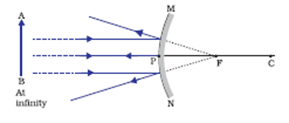(ii) When object is placed between pole and infinity

Image Position – Between ‘P’ and ‘F’

Nature of image – Virtual, erect

Size – Diminished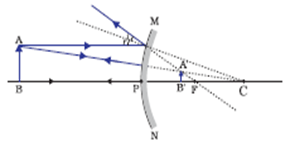A full length image of a tall building/tree can be seen in a small convex mirror

Uses of Convex Mirror

• Convex mirrors are used as rear view mirrors in vehicles because

– they always give an erect though diminished image

– they have a wider field of view as they are curved outwards

• Convex mirrors are used at blind turns and on points of merging traffic to facilitate vision of both side traffic
• Used in shops as security mirror

Sign Convention for Reflection by Spherical Mirror

• The object is placed to the left of the mirror
• All distances parallel to the principal axis are measured from the pole of the mirror
• All distances measured in the direction of incident ray (along + X-axis) are taken as positive and those measured against the direction of incident ray (along – X-axis) are taken as negative
• Distance measured perpendicular to and above the principal axis are taken as positive
• Distances measured perpendicular to and below the principal axis are taken as negative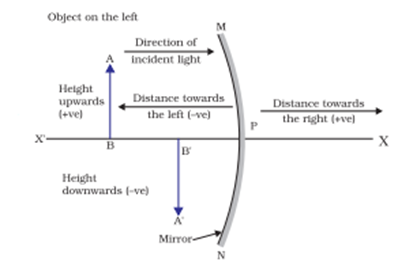• Object distance = ‘u’ is always positive
• Focal length of concave mirror = negative
• Focal length of convex mirror = positive

Mirror Formula

1/v + 1/u = 1/f

Where v=image distance

u= object distance

f=Focal length

Magnification of Spherical Mirrors

It is the ratio of the height of the image to the height of the object

m=Height of image/Height of the object

=> m = hi/ho

Also, m = -v/u

• If ‘m’ is negative, image is real
• If ‘m’ is positive, image is virtual
• If ‘hi‘ = ho then m = 1, i.e., image is equal to object
• If hi > ho then m > 1 i.e., image is enlarged
• If hi < ho then m < 1 i.e., image is diminished

Magnification of plane mirror is always + 1

‘1’ indicates that image is equal to object’s size

• If ‘m’ is positive and less than 1, it is a convex mirror
• If ‘m’ is positive and more than 1, it is a concave mirror
• If ‘m’ is negative, it is a concave mirror

## Refraction

The natural phenomenon of changing the path of light while moving from one medium to another is termed as the Refraction of light.

As the light travels from its rarer to a denser medium, light rays bend towards normal and while travelling from the denser to a rarer medium, light rays bend away from normal. According to the law of Refraction:

The Refraction of light occurs when there is a change in the speed of light and when the light rays enter from one transparent medium to another. Speed of light is maximum in vacuum. It is 3 x 108 m/s. Cause of refraction is a change in the speed of light.

Some examples of refraction

• The bottom of swimming pool appears higher
• A pencil partially immersed in water appears to be bent at the interface of water and air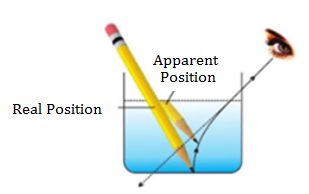• Lemons placed in a glass tumbler appear bigger
• Letters of a book appear to be raised when seen through a glass slab

Refraction takes place when light strikes on a polished surface like mirror and bounces back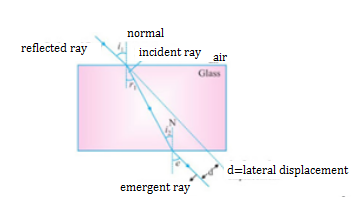• The extent of bending of ray of light at the opposite parallel faces of rectangular glass slab is equal and opposite, so the ray emerges parallel to incident ray
• Lateral displacement depends on

– refractive index of glass slab

– thickness of the glass slab

Laws Of Refraction

• The incident ray, the reflected ray and the normal to the interface of two transparent media at the point of incidence, all lie in the same plane
• Snell’s law – the ratio of sine of angle of incidence to the sine of angle of refraction is a constant, for a light of given colour and for a given pair of media

sin i / sin r = constant

Refractive index (n) – The ratio of speed of light in a given pair of media

n = Velocity of light in medium 1/Velocity of light in medium 2

-> n21 means refractive index of second medium with respect to first medium

n21 = v1/v2

-> n12 means refractive index of first medium with respect to second medium

n12 = v2/v1

Absolute Refractive Index

It is the refractive index of a medium with respect to vacuum or air.

n= (c/v) x c = 3 x 108 m/s

The refractive index of one medium is reciprocal of other’s refractive index in a given pair. n12 = 1/ n21

If the refractive index of medium 1 w.r.t air is given as 1nair and if the refractive index of medium 2 w.r.t. air is given as 2nair . Then the refractive index of medium 1 w.r.t. medium 2 is given by (1nair ) / (1nair )

Refractive index of diamond is highest till date. It is 2.42. It means the speed of light is 1/2.42 times less in diamond than in vacuum.

Optically denser medium – out of two given media, the medium with a higher value of the refractive index

Optically rarer medium – Out of two given media, the medium with lower value to refractive index

When light enters obliquely from a rarer to a denser medium, it bends towards the normal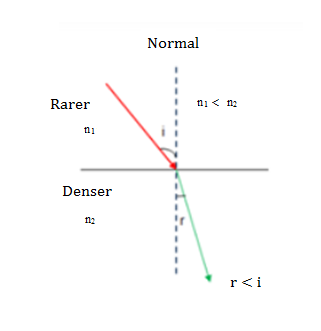When light enters obliquely from a denser to a rarer medium, it bends away from the normal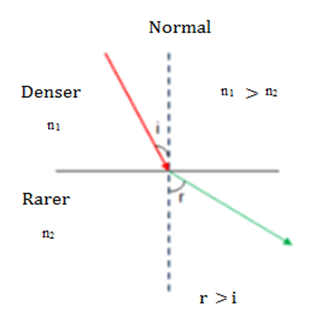The refractive index of a medium does not depend on physical density.

Spherical Lens – A transparent medium bound by two surfaces, of which one or both surfaces are curved.

 Convex lens Concave lens Thin from corners Thick from corners Thick at centre Thin at centre Converging Diverging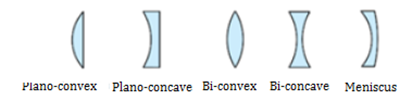Rules for image formation by Convex Lens

(i) A ray of light parallel to the principal axis of a convex lens always pass through the focus on the other side of the lens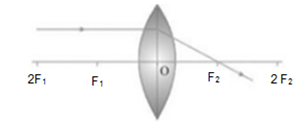(ii) A ray of light passing through the principal focus will emerge parallel to principal axis after refraction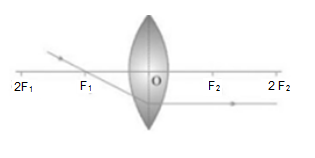(iii) A ray of light passing through the optical centre will emerge without any deviation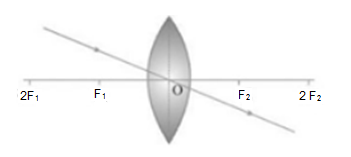Ray Diagrams of Image formed by Convex Lens

(i) When object is at infinity

Image position – At ‘F2’

Nature of image – Real, inverted

Size – Point sized or highly diminished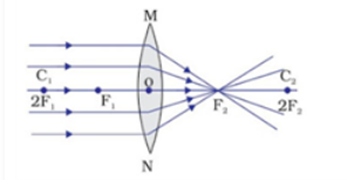(ii) When object is beyond ‘2F1’

Image position – Between ‘F2’ and ‘2F2’

Nature of image – Real, inverted

Size – Diminished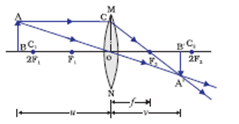(iii) When object is at ‘2F1’

Image position – At ‘2F2’

Nature of image – Real, inverted

Size – Same size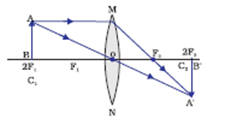(iv) When object is between ‘F1’ and ‘2F1’

Image position – Beyond ‘2F2’

Nature of image – Real, inverted

Size – Enlarged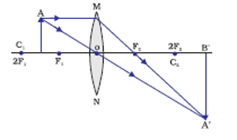(v) When object is at ‘F1’

Image position – At infinity

Nature of image – Real, inverted

Size – Highly Enlarged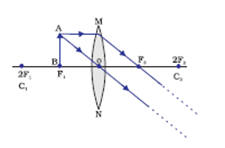(vi) When object is between ‘F1’ and optical centre

Image position – On the same side of the lens as object

Nature of image – Virtual and erect

Size – Enlarged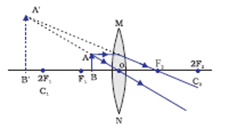Rules for Image formation by Concave Lens

(i) A ray of light parallel to the principal axis appears to diverge from the principal focus located on the same side of the lens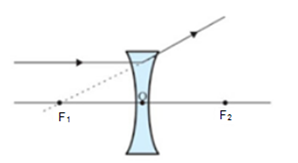(ii) A ray of light appearing to meet at the principal focus of a concave lens will emerge parallel to the principal axis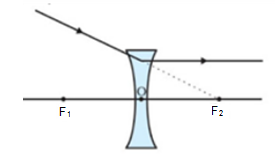(iii) A ray of light passing through the optical centre of a lens will emerge without any deviation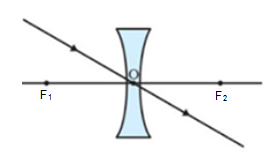Ray Diagrams Of Images Formed By A Concave Lens

(i) When object is placed at infinity

Image position – At ‘F1’

Nature of image – Virtual and erect

Size – Point sized or highly diminished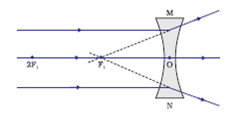(ii) When object is placed between infinity and optical centre

Image position – between ‘F’ and ‘O’

Nature of image – Virtual and erect

Size – Diminished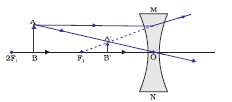Sign Convention For Spherical Lenses

• Sign conventions are similar to the one used for spherical mirrors, except the measurements are taken from optical centre of the lens
• Focal length of convex lens = Positive

Focal length of concave lens = Negative

Lens Formula

1/v – 1/u = 1/f

Magnification

M = hi/ho = v/u

Power of Lens

• It is defined as the reciprocal of focal length in meter
• The degree of convergence or divergence of light rays is expressed in terms of power

Power(P) = 1/v – 1/u = 1/f

• S.I. unit of Power = Dioptre = D
• 1 D = 1 m-1
• 1 dioptre is the power of lens whose focal length is one metre
• Power of convex lens = positive
• Power of concave lens = negative
• Power = 1/(focal length or thickness)
• Power of a lens combination (P) = P1 + P2 + P3

Few Important Questions

• Difference between Reflection and Refraction of light.
• Difference between Concave mirrors and Convex mirrors.
• What is a spherical mirror? List out the uses of spherical mirrors.
 NCERT Related Articles NCERT Solutions for Class 12 NCERT Solutions for Class 11 NCERT Solutions for Class 10 NCERT Solutions for Class 12 Maths NCERT Solutions for Class 11 Maths NCERT Solutions for Class 10 Maths NCERT Solutions for Class 12 Physics NCERT Solutions for Class 11 Physics NCERT Solutions for Class 10 Science NCERT Solutions for Class 12 Chemistry NCERT Solutions for Class 11 Chemistry NCERT Solutions for Class 6 NCERT Solutions for Class 12 Biology NCERT Solutions for Class 11 Biology NCERT Solutions for Class 6 Maths NCERT Solutions for Class 9 NCERT Solutions for Class 8 NCERT Solutions for Class 6 Science NCERT Solutions for Class 9 Maths NCERT Solutions for Class 8 Maths NCERT Solutions for Class 4 NCERT Solutions for Class 9 Science NCERT Solutions for Class 8 Science NCERT Solutions for Class 4 Maths NCERT Solutions for Class 5 NCERT Solutions for Class 7 NCERT Solutions for Class 7 Maths NCERT Solutions for Class 4 Science NCERT Solutions for Class 5 Maths NCERT Solutions for Class 7 Science NCERT Solutions NCERT Books NCERT Solutions for Class 5 Science